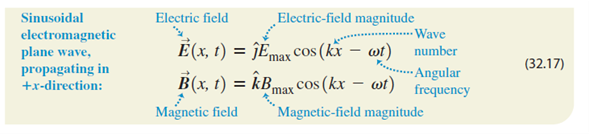# Problem: A sinusoidal electromagnetic wave having a magnetic field of amplitude 1.25 μT and a wavelength of 432 nm is traveling in the +-direction through empty space.Write the equations for the electric and magnetic fields as functions of x and t in the form of Eqs. (32.17).

###### FREE Expert Solution

Wave number:

$\overline{){\mathbf{k}}{\mathbf{=}}\frac{\mathbf{2}\mathbf{\pi }}{\mathbf{\lambda }}}$

Angular frequency:

$\overline{){\mathbf{\omega }}{\mathbf{=}}{\mathbf{2}}{\mathbf{\pi }}{\mathbf{f}}}$

k = 2π/λ = 2π/432 × 10-9  = 1.45 × 107 rad/m

1/λ = k/2π

ω = 2πf = 2π(c/λ) = 2π(ck/2π) = ck = 3.0 × 108 × 1.45 × 107  = 4.35 × 1015 rad/s

83% (46 ratings)###### Problem Details

A sinusoidal electromagnetic wave having a magnetic field of amplitude 1.25 μT and a wavelength of 432 nm is traveling in the +-direction through empty space.

Write the equations for the electric and magnetic fields as functions of x and t in the form of Eqs. (32.17).Frequently Asked Questions

What scientific concept do you need to know in order to solve this problem?

Our tutors have indicated that to solve this problem you will need to apply the Electromagnetic Waves as Sinusoidal Waves concept. You can view video lessons to learn Electromagnetic Waves as Sinusoidal Waves. Or if you need more Electromagnetic Waves as Sinusoidal Waves practice, you can also practice Electromagnetic Waves as Sinusoidal Waves practice problems.

What professor is this problem relevant for?

Based on our data, we think this problem is relevant for Professor Chini's class at UCF.